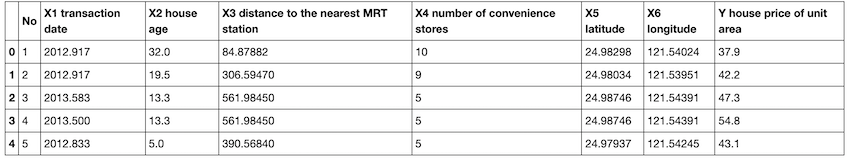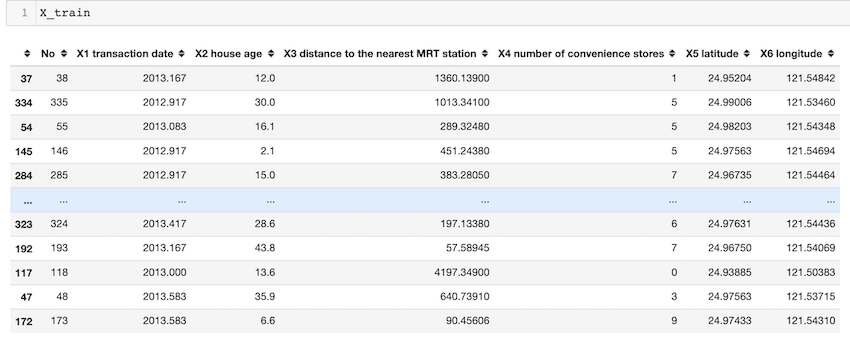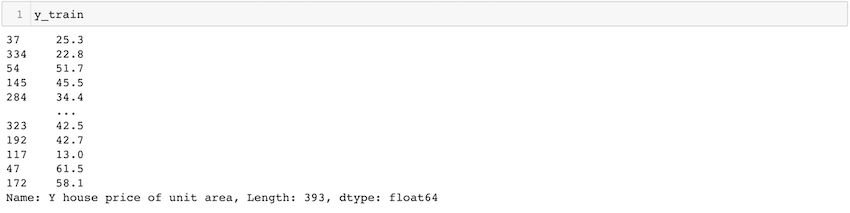# How to split a Dataset into Train and Test Sets using Python

• Last Updated : 09 Feb, 2022

In this article, we will discuss how to split a dataset into Train and Test sets in Python.

The train-test split is used to estimate the performance of machine learning algorithms that are applicable for prediction-based Algorithms/Applications. This method is a fast and easy procedure to perform such that we can compare our own machine learning model results to machine results. By default Test set is split into 30 % of actual data and the Training set is split into 70% of the actual data

We need to split a dataset into train and test sets to evaluate how well our machine learning model performs. The train set is used to fit the model, the statistics of the train set are known. The second set is called the test data set, this set is solely used for predictions.

## Dataset Splitting:

Scikit-learn alias sklearn is the most useful and robust library for machine learning in Python.

The scikit-learn library provides us with the model_selection module in which we have the splitter function train_test_split().

Syntax:

train_test_split(*arrays, test_size=None, train_size=None, random_state=None, shuffle=True, stratify=None)

parameters:

1. *arrays : inputs such as lists, arrays, dataframes or matrices
2. test_size : this is a float value whose value ranges between 0.0 and 1.0. it represents the proportion of our test size. it’s default value is none.
3. train_size : this is a float value whose value ranges between 0.0 and 1.0. it represents the proportion of our train size. it’s default value is none.
4. random_state: this parameter is used to control the shuffling applied to the data before applying the split. it acts like a seed.
5. shuffle: This parameter is used to shuffle the data before splitting. it’s default value is true.
6. stratify: This parameter is used to split the data in stratified fashion.

### Example:Code:

## Python3

 `# import modules` `import` `pandas as pd` `from` `sklearn.linear_model ``import` `LinearRegression`   `# read the dataset` `df ``=` `pd.read_csv(``'Real estate.csv'``)`   `# get the locations` `X ``=` `df.iloc[:, :``-``1``]` `y ``=` `df.iloc[:, ``-``1``]`   `# split the dataset` `X_train, X_test, y_train, y_test ``=` `train_test_split(` `    ``X, y, test_size``=``0.05``, random_state``=``0``)`

In the above example, We import the pandas package and sklearn package. after that to import the CSV file we use read_csv() method. The variable df now contains the data frame. in the example “house price” is the column we’ve to predict so we take that column as y and the rest of the columns as our X variable. test_size = 0.05 specifies only 5% of the whole data is taken as our test set, and 95% as our train set. The random state helps us get the same random split each time.

Output:My Personal Notes arrow_drop_up
Recommended Articles
Page :